• 进制转换器项目要求：用户提供需要转换的数据和该数据的进制，以及要转换的进制，进制转换器提供给用户最终的正确转换的结果。 转换器实例 例如，用户提供了一个十进制数：10，要求将此数据以二进制形式转换，则...
进制转换器项目要求：用户提供需要转换的数据和该数据的进制，以及要转换的进制，进制转换器提供给用户最终的正确转换的结果。
转换器实例
例如，用户提供了一个十进制数：10，要求将此数据以二进制形式转换，则通过进制转换器转换的最终结果应该：1010。
提示：此进制转换器可以在
2-36 进制之间对数据进行任意转换。各进制中对应的数字如下表：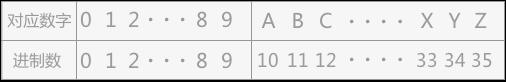设计思路 当用户给定 2 - 36 进制中的任意一进制数时，最简单的方法是使用顺序存储结构进行存储，即使用字符串数组存储。
转化时，最直接的思路就是先将该数转化为十进制数据，然后再由十进制转化成要求的进制数，最终的结果用栈结构存储（先进后出），这样最终显示 给用户的是正常的数据。
实现代码
#include <stdio.h>
#include <string.h>
#include <math.h>
int top=-1;//top 变量时刻表示栈顶元素所在位置
void push(char * a,char elem){
a[++top]=elem;
}
void pop(char * a){
if (top==-1) {
return ;
}
//输出时要按照正确的格式显示给用户
if (a[top]>=10) {
printf("%c",a[top]+55);
}else{
printf("%d",a[top]);
}
top--;
}

//将各进制数转换成十进制数
int scaleFun(char * data,int system){
int k=(int)strlen(data)-1;
int system_10_data=0;
int i;
for (i=k; i>=0; i--) {
int temp;
if (data[i]>=48 && data[i]<=57) {
temp=data[i]-48;
}else{
temp=data[i]-55;
}
system_10_data+=temp*pow(system, k-i);
}
return system_10_data;
}
int main() {
char data;
printf("进制转换器，请输入原数据的进制（2-36）:");
int system;
scanf("%d",&system);
getchar();
printf("请输入要转换的数据：");
scanf("%s",data);
getchar();
int system_10_data=scaleFun(data, system);
printf("请输入转换后的数据的进制：");
int newSystem;
scanf("%d",&newSystem);
getchar();
while (system_10_data/newSystem) {
push(data,system_10_data%newSystem );
system_10_data/=newSystem;
}
push(data,system_10_data%newSystem);
printf("转换后的结果为：\n");
while (top!=-1) {
pop(data);

}
}

展开全文c++ c语言 算法 经验分享
• C++语言实现带小数的任意进制转换，使用了数据结构中的栈和队列，在VC++6.0上编译运行通过。对于学习C++和数据结构有一定的参考意义！
• 符合进制转换的方式，故用栈这种结构。 一.定义声明 #include<iostream> using namespace std; #define OK 1 #define ERROR 0 typedef int Status; typedef int ElemType; //定义结构体： typedef struct ...
为什么要用栈这种结构在十进制转换为其他进制时，先算的余数由下而上组成的数是最终转换结果，见上图。而栈这种结构中，元素先进后出。符合进制转换的方式，故用栈这种结构。
一.定义声明
#include<iostream>
using namespace std;
#define OK 1
#define ERROR 0
typedef int Status;
typedef int ElemType;
//定义结构体：
typedef struct Node
{
ElemType data;
struct Node   * next;
//函数的声明：
Status InitStack ( LinkStack   &s );
Status   Push ( LinkStack   &s , ElemType  e );
Status   Pop ( LinkStack   &s , ElemType  &e );
bool   StackEmpty ( LinkStack   s );
void Conversion(int x,  int r);//x：转换数，r：进制

二.主函数
int main()
{int x,r,T;
while(1)
{
cout<<"输入转换数和进制:";
cin>>x>>r;
if(x==0||r==0)//如果x==0或者r==0退出循环
{
break;
}
else
{
Conversion( x,   r);
printf("\n");
}
}
}

三.函数部分
Status InitStack ( LinkStack   &s )
{
s=new StackNode;
if(!s) return ERROR;
s->next==NULL;
return OK;
}
//入栈
Status   Push ( LinkStack   &s , ElemType  e )
{
StackNode *p=new StackNode;
p->data=e;
p->next=s->next;
s->next=p;
return OK;
}
//出栈
Status   Pop ( LinkStack   &s , ElemType  &e )
{
StackNode *p;
if(s->next==NULL) return ERROR;
p=s->next;
e=p->data;
s->next=p->next;
delete p;
return OK;
}
bool   StackEmpty ( LinkStack   s )
{
return  (s->next==NULL);
}

void Conversion(int x,  int r)   //x：转换数，r：进制
{
InitStack(s);
while  ( x )
{
Push ( s, x % r ) ;
x=x / r ;
}
while ( ! StackEmpty(s) )
{
Pop(s,e);
cout<<e;
}
}

四.说明
while  ( x )
{
Push ( s, x % r ) ;
x=x / r ;
}

关于对上述代码的解释： x%r的值（值为x➗r的余数）入栈 十进制转二进制 以202为例。202对先2进行除法运算得出的余数记录在右侧比如:202/2=101余0，用101继续进行除2运算101/2=50余…最后结果从下到上读取:11001010。展开全文c++
• printf("进制转化，请输入原数据进制（2-36）："); int system; scanf("%d", &system); getchar(); printf("请输入要转化的数据："); scanf("%s", data); getchar(); int system_10_data = scanfFun...
代码：
#include<stdio.h>
#include<string.h>
#include<math.h>
int top = -1;//栈的初始化-代表空栈
void push(char *a, char elem) {//入栈
a[++top] = elem;
}
void pop(char *a) {
if (top == -1) {
return;
}
if (a[top] >= 10) {
printf("%c", a[top] + 55);
}
else
{
printf("%d", a[top]);
}
top--;
}
//将各进制数转化成十进制数
int scanfFun(char *data, int system) {
int k = (int)strlen(data) - 1;
int system_10_data = 0;
int i;
for (i = k; i > 0; i++) {
int temp;
if (data[i] > 48 && data[i] <= 57) {
temp = data[i] - 48;
}
else
{
temp = data[i] - 55;
}
system_10_data += temp * pow(system, k - i);
}
return system_10_data;
}

int main() {
char data;//定义了一个数组100长度
printf("进制转化器，请输入原数据的进制（2-36）：");
int system;
scanf("%d", &system);
getchar();

printf("请输入要转化的数据：");
scanf("%s", data);
getchar();

int system_10_data = scanfFun(data, system);
printf("请输入转化后的数据进制：");
int newSystem;
scanf("%d", newSystem);
getchar();

while (system_10_data / newSystem) {
push(data, system_10_data%newSystem);
system_10_data /= newSystem;
}
push(data, system_10_data%newSystem);
printf("转换后的结果为：\n");
while (top != -1) {
pop(data);
}

}

结果：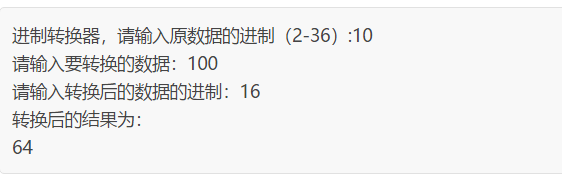有点问题
展开全文• 代码很简单，功能也很简单，这里就不多... printf("请输入一个十进制数，我能帮您把它转换成2~16任意进制数：\n"); scanf("%d",&y); b=y; printf("请输入要转换为的进制:"); scanf("%d",&w); printf("\n"); do {
• ## 数据结构练习——栈（进制转换）

千次阅读 多人点赞 2018-08-18 15:39:39
看完小王八老师的数据结构栈的课程，我觉得有必要联系进制转换，写下来记录一下。 数据结构练习——栈（进制转换）C语言实现 首先模拟栈 使用字符串模拟栈，用动态创建的方法模拟栈结构比较好，但是我是数据结构...
看完小王八老师的数据结构栈的课程，我觉得有必要联系进制转换，写下来记录一下。
数据结构练习——栈（进制转换）C语言实现
首先模拟栈
使用字符串模拟栈，用动态创建的方法模拟栈结构比较好，但是我是数据结构小白，所以见笑了。
下面贴上我的c工程里的头文件中栈的代码：
//定义栈
struct Stack
{
char mData;
int mLen;
};

//初始化栈
void InitStack(struct Stack *S)
{
S->mLen = -1;
}
//元素入栈
void Push(struct Stack *S,char item)
{
S->mData[++S->mLen] = item;
}
//元素出栈
char Pop(struct Stack *S)
{
return S->mData[S->mLen--];
}
//返回栈顶元素
char  Top(struct Stack *S)
{
return S->mData[S->mLen];
}
//清空栈
void Clear(struct Stack *S)
{
int i;
for(i = 0;i<=S->mLen;i++)
{
Pop(S);
}
}
//是否为空 是空返回1 否则返回0
int StackEmpty(struct Stack *S)
{
if(S->mLen == -1)
return 1;
else
return 0;
}


接下来贴图说明一下，几种进制转换实现方法：
十进转八进：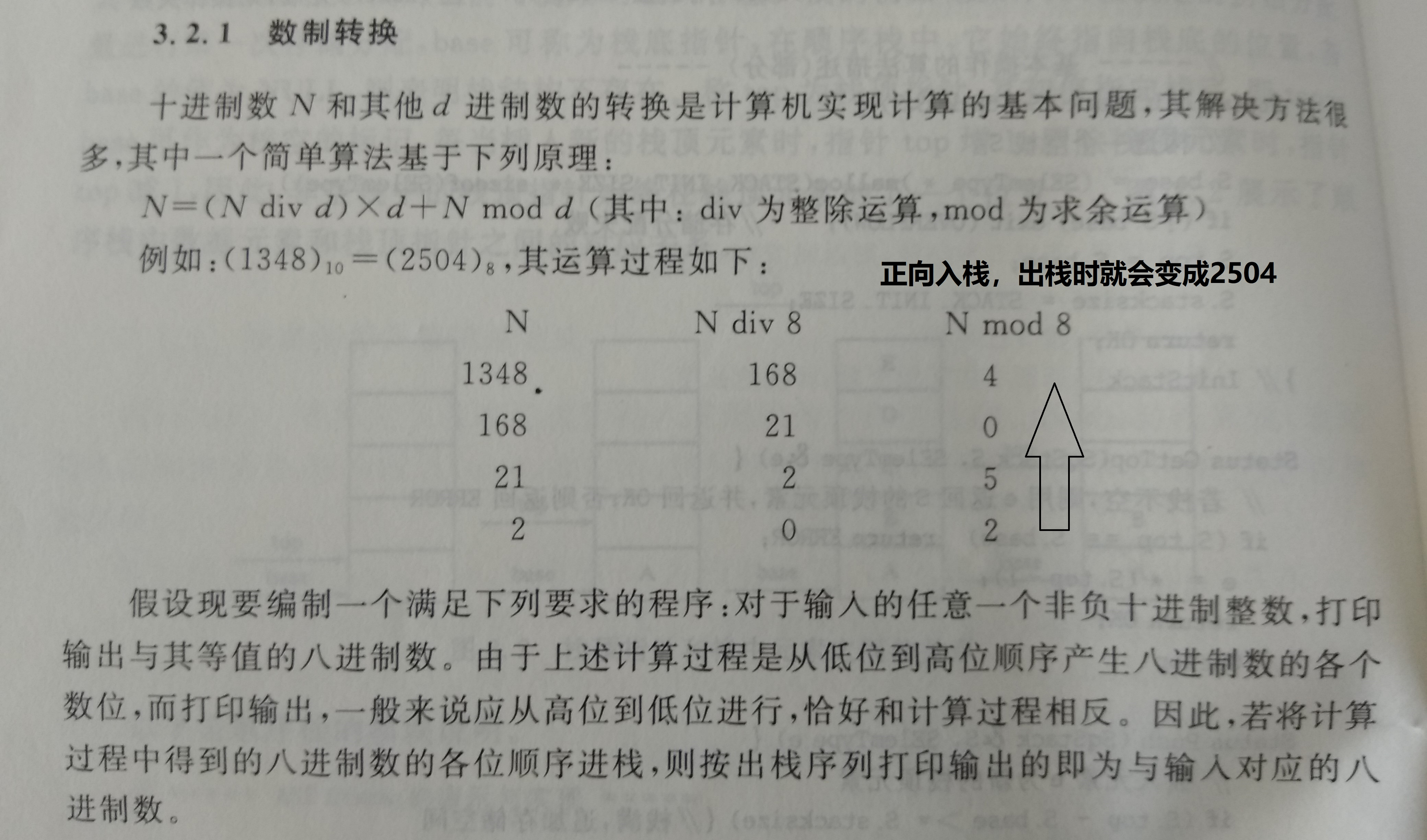void converse_Dec_to_Oct()   //十进制转八进制
{
int N;
char e;
struct Stack S;
InitStack(&S);
printf("Decimal to Octonary(8) please input data:\n");
scanf("%d",&N);
//转换
while(N)
{
Push(&S,(char)(N%8+48));
N = N/8;
}
//输出
printf("converse:");
while(!StackEmpty(&S))
{
e = Pop(&S);
printf("%c",e);
}

}
二进制转十进制：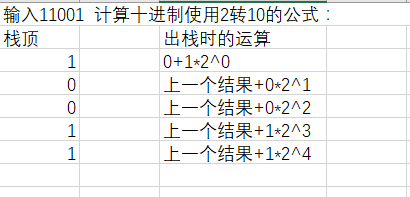void converse_bin_to_Dec()  //二进制转换十进制，使用一个栈
{
struct Stack s;
InitStack(&s);
char str;
int outcome=0;    //记录结果
int i;

printf("binary to Decimal(10) please input data:\n");
scanf("%s",str);
// 压入栈
for(i=0;i<strlen(str);i++)
{
Push(&s,str[i]);
}
//转换
for(i=0;!StackEmpty(&s);i++)
{
outcome += (int)(Pop(&s)-48)*pow(2,i);  //字符转int
}
//输出
printf("converse:");
printf("%d",outcome);
}
二进制转换八进制：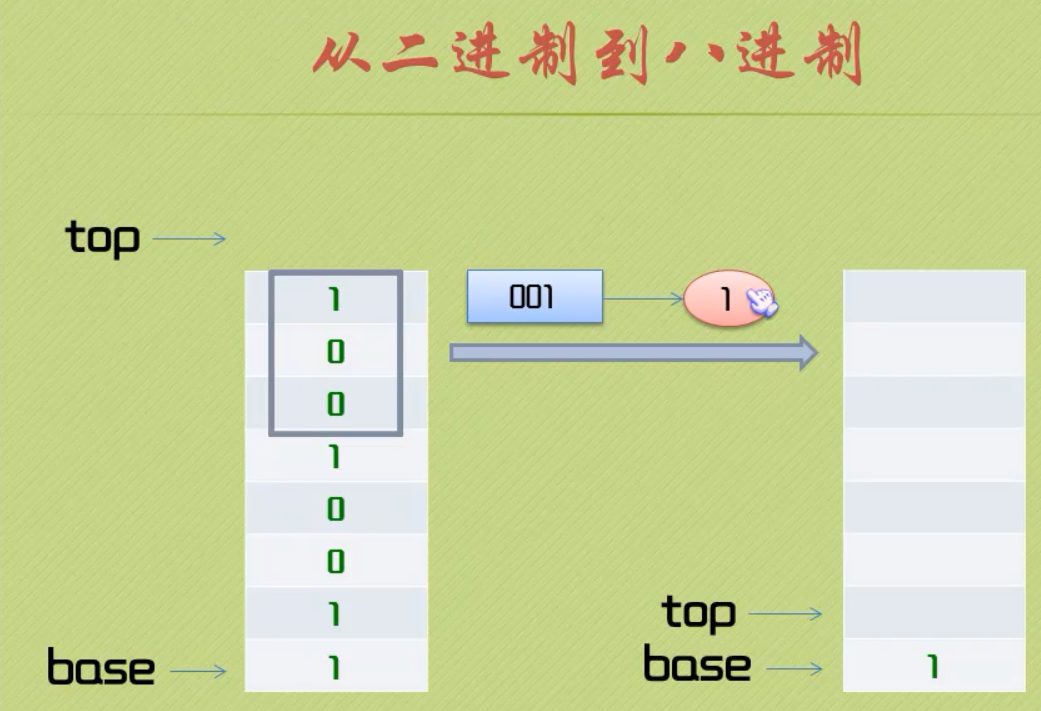可以看到，二进制的3位转换为8进制的1位，所以将二进制串按照3为一个组，转换为8进制的一位压入8进制栈中。
而且此处体现出栈的特点FILO（First In Last Out），转换完成后输出8进制栈的时候正好是各位顺序正确的8进制数。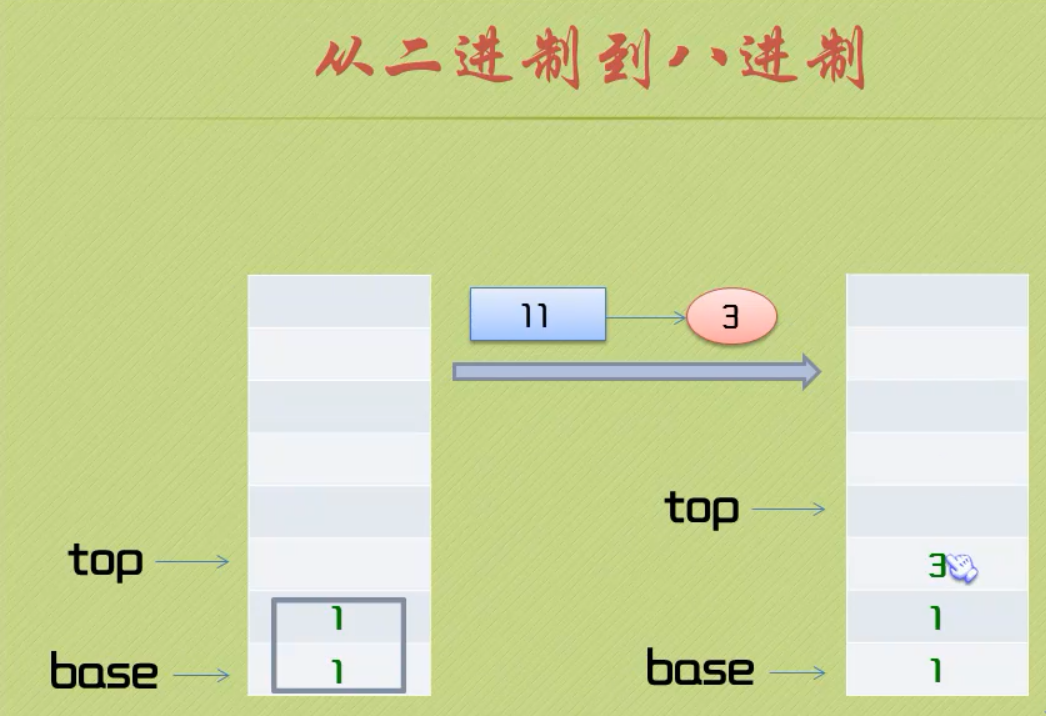注意有时候会有不足二进制栈剩余三位的情况，需要稍微处理一下，上代码：
void converse_bin_to_Oct()  //二进制转换八进制，使用两个栈
{
struct Stack s,t;
int temp=0;
char str;
InitStack(&s);InitStack(&t);
int i;

printf("binary to octonary(8) please input binary data:\n");
scanf("%s",str);
// binary元素压入栈
for(i=0;i<strlen(str);i++)
{
Push(&s,str[i]);
}
//转换
while(!StackEmpty(&s))
{
temp=0; //每次循环执行时temp置0
for(i=0;i<3&&!StackEmpty(&s);i++)   //当读取三位binary或者binary栈为空时跳出
temp += (int)(Pop(&s)-48)*pow(2,i);

Push(&t,(char)(temp+48));  //转换后的8进制数压入8进制栈
}
//输出8进制栈
printf("converse:");
while(!StackEmpty(&t))
{
printf("%c",Pop(&t));
}
}
二进制转换十六进制：
原理和8进制一样，不赘述了，但是注意十六进制使用A-F表示10-15：
void converse_bin_to_Hex()  //二进制转换十六进制，使用两个栈
{
struct Stack s,t;
int temp=0;
char str;
InitStack(&s);InitStack(&t);
int i;

scanf("%s",str);
// binary元素压入栈
for(i=0;i<strlen(str);i++)
{
Push(&s,(int)(str[i]-48));
}
//转换
while(!StackEmpty(&s))
{
temp=0; //每次循环执行时temp置0
for(i=0;i<4&&!StackEmpty(&s);i++)   //当读取三位binary或者binary栈为空时跳出
temp += Pop(&s)*pow(2,i);
if(temp<=9)
Push(&t,(int)(temp+48));  //转换后的8进制数压入8进制栈
else
{
switch(temp)
{
case 10:Push(&t,'A');break;
case 11:Push(&t,'B');break;
case 12:Push(&t,'C');break;
case 13:Push(&t,'D');break;
case 14:Push(&t,'E');break;
case 15:Push(&t,'F');break;
}
}
}

//输出16进制栈
printf("converse:");
while(!StackEmpty(&t))
{
printf("%c",Pop(&t));
}
}

下面贴上整个main.c代码，头文件在上面贴出来了。
#include <stdio.h>
#include <stdlib.h>
#include <math.h>
#include <string.h>

// 栈使用字符串模拟
void converse_Dec_to_Oct()   //十进制转八进制
{
int N;
char e;
struct Stack S;
InitStack(&S);
printf("Decimal to Octonary(8) please input data:\n");
scanf("%d",&N);
//转换
while(N)
{
Push(&S,(char)(N%8+48));
N = N/8;
}
//输出
printf("converse:");
while(!StackEmpty(&S))
{
e = Pop(&S);
printf("%c",e);
}

}

void converse_bin_to_Dec()  //二进制转换十进制，使用一个栈
{
struct Stack s;
InitStack(&s);
char str;
int outcome=0;    //结果
int i;

printf("binary to Decimal(10) please input data:\n");
scanf("%s",str);
// 压入栈
for(i=0;i<strlen(str);i++)
{
Push(&s,str[i]);
}
//转换
for(i=0;!StackEmpty(&s);i++)
{
outcome += (int)(Pop(&s)-48)*pow(2,i);  //字符转int
}
//输出
printf("converse:");
printf("%d",outcome);
}

void converse_bin_to_Oct()  //二进制转换八进制，使用两个栈
{
struct Stack s,t;
int temp=0;
char str;
InitStack(&s);InitStack(&t);
int i;

printf("binary to octonary(8) please input binary data:\n");
scanf("%s",str);
// binary元素压入栈
for(i=0;i<strlen(str);i++)
{
Push(&s,str[i]);
}
//转换
while(!StackEmpty(&s))
{
temp=0; //每次循环执行时temp置0
for(i=0;i<3&&!StackEmpty(&s);i++)   //当读取三位binary或者binary栈为空时跳出
temp += (int)(Pop(&s)-48)*pow(2,i);

Push(&t,(char)(temp+48));  //转换后的8进制数压入8进制栈
}
//输出8进制栈
printf("converse:");
while(!StackEmpty(&t))
{
printf("%c",Pop(&t));
}
}

void converse_bin_to_Hex()  //二进制转换十六进制，使用两个栈
{
struct Stack s,t;
int temp=0;
char str;
InitStack(&s);InitStack(&t);
int i;

scanf("%s",str);
// binary元素压入栈
for(i=0;i<strlen(str);i++)
{
Push(&s,(int)(str[i]-48));
}
//转换
while(!StackEmpty(&s))
{
temp=0; //每次循环执行时temp置0
for(i=0;i<4&&!StackEmpty(&s);i++)   //当读取三位binary或者binary栈为空时跳出
temp += Pop(&s)*pow(2,i);
if(temp<=9)
Push(&t,(int)(temp+48));  //转换后的8进制数压入8进制栈
else
{
switch(temp)
{
case 10:Push(&t,'A');break;
case 11:Push(&t,'B');break;
case 12:Push(&t,'C');break;
case 13:Push(&t,'D');break;
case 14:Push(&t,'E');break;
case 15:Push(&t,'F');break;
}
}
}

//输出16进制栈
printf("converse:");
while(!StackEmpty(&t))
{
printf("%c",Pop(&t));
}
}

int main()
{
int choose=0;
printf("which conversion do want to choose:\n10 ot 8:1\n2 to 10:2\n2 to 8: 3\n2 to 16:4\n\ninput: ");
scanf("%d",&choose);
fflush(stdin);
switch(choose)
{
case 1:converse_Dec_to_Oct();
case 2:converse_bin_to_Dec();
case 3:converse_bin_to_Oct();
case 4:converse_bin_to_Hex();
}

printf("\nHello world!\n");
return 0;
}

数据结构小白，有不对的地方，请大佬指出，谢谢！

展开全文• 进制转换一直是保研机试的时候的热门考点，本文来介绍进制转换的处理方法。c++
• 虽然Java开发者已经对其进行了封装，但还是要学会自己编写相应的进制转换器的。 常用的进制有二进制，八进制，十进制，十六进制。 二.将整数转换成十六进制 二.一 将int整数转换成十六进制（一） 先写一个主方法，...
• 写一个程序，将输入的十进制数据M 转换为八进制数据M8，将其调试通过。 在此基础上修改程序，实现十进制数据M 向N 进制（2或8或16）的转换。   （1）采用顺序存储结构实现栈。 #include&lt;stdio.h&gt...
• 利用栈实现进制转换，支持16进制以下进制（2-10、16），更改相应的参数还可扩展其他进制转换，还可以通过更改宏定义来扩宽进制转换数据范围。用户通过输入想要转换的进制和待转换的数字
• 将各个进制转换重新整理了以下，用栈的灵活机制写了一个进制转换器。图：代码：Stack.h#ifndef _STACK_H_ #define _STACK_H_ #include &lt;stdbool.h&gt; #define STACK_INIT_SIZE 100 //栈控件初始化大小 ...
• 1.很久没有更新博客了 这次想要记录分享一下在这段学习过程中实现的进制转换器 c语言代码 里面运用到了一些数据结构和递归的相关知识 也算是一个简单的综合运用吧 2.核心思想是将一切进制转换为10进制 再由十进制...
• 我的其他资源都是免费的，是对于c语言初学者的帮助比较大的，其中有单片机，ARM，数据结构，window编程，MFC编程，自己编写的小游戏。
• 把一个十进制整数转换为2至9进制之间的任意进制数输出
• 进行数制转换这类运算最简单的方法就是使用栈的数据结构。 （1）在输入二进制数时，将每次输入的0/1压入栈A中保存。 （2）转换时，要将二进制的每三位转换成八进制的一位，先得到的是八进制的低位数，因此可以将八...
• 进制转换器项目要求：用户提供需要转换的数据和该数据的进制，以及要转换的进制，进制转换器提供给用户最终的正确转换的结果。 转换器实例 例如，用户提供了一个十进制数：10，要求将此数据以二进制形式转换，则...
• ## 进制转换心得

千次阅读 2016-05-06 09:25:06
1.首先判断要转换数值的大小  i nt 2147483648～2147483647  long long的最大值：9223372036854775807  long long的最小值：-...2.N进制转换到M进制（N、M不等于10）  先转换到十进制，再进行下一步转换。
• //进制转换函数 void xiao(Pe *S); //销毁栈 void chu(Pe S); //出栈函数 int main() { Pe S; int x,y; printf("输入要转换的十进制数和要转换的进制：\n"); scanf("%d %d",&x,&y); S=zhuan(x,y); chu(S); ...c语言
• 万能进制转换器，能转换到意想不到的进制(⊙o⊙) —— 数据结构中栈的应用 ——对应教材上算法的扩展算法 c++
• 进制转换算法概念 其核心是利用栈的存储结构性质，进行数据的入栈出栈时的计算，让后将计算好的数据存入另一个栈内，最后再出栈输出。由于栈的先进后出特性，最后输出的顺序和输入的顺序是一样的。具体如上图。 ...
• 最近在学习java语言的基础，用学到的基础知识做了一个进制转化，分享一下 package day1; import java.util.Scanner; public class Conversion { static int from;//待转换进制 static int to;//转换后的...java
• 把十进制转换为2进制。使用方法为： dec2bin(A)；则自动把A转换为2进制数据。 dec2bin(A,minDigitals);则把A转换为minDigitals位宽的数据。高位不足的补0；如果minDigitals小于A所需要的位宽，则转换的2进制数据为...
• 我们都熟悉任意进制和十进制之间的转换，任意进制转换的思路就是以十进制为桥梁，先把数字转成十进制，再把十进制转成目的进制。 任意进制转十进制，比如：45(8)，转成十进制，其 result = 4x81+5x80 = 32+5 = 37...
• # 使用顺序栈实现十进制转换成其他进制操作 class sstack(): def __init__(self): # 初始化一个空栈 self.slist= [] def is_empty(self):# 判断栈是否为空 if self.slist == []: return 1 else: return 0 ...
• 这个题目作为栈的开始，我们需要先了解一下栈，栈包括栈顶，栈底，栈顶指示，空栈，满栈，上溢，下溢等概念，栈是一种特殊的一种顺序表，栈的特性是后进先出，并且不能插队进栈出栈。栈的基本操作还是比较容易的，...
• - 数据解释, 已知 20 种数据类型 - 使用模板编辑数据结构 (例如: 修复分区表/引导扇区) - 连接和分割、以奇数偶数字节或字的方式合并、分解文件 - 分析和比较文件 - 搜索和替换功能尤其灵活 - 磁盘克隆 (可在 DOS ...
• 【源代码】C++算法（四）队列的基本操作 （用队列实现十进制转换十六进制）
• 我这边需要换算 16进制 的 12 十六进制已开头 0x 表示 计算机表达 0x12 ^表示次方 计算公式: 拆分数字 * 16 ^ (数字位置-1)  如何进行去换算。 单独把 12 拎出来 12 的十六进制= 1*16^1 + 2*16^0 = 16 + 2 =...
•  这是一个利用栈实现的实例，自己写了一下，本想编写成各个进制之间都可以相互转换的“转换器”，可是在最开始的地方就已经卡住了，主要卡住的地方就是如何让用户自己输入一串10101代码(不需要限制)，从而实现转换...c语言
• 　由于计算机的特殊结构，其内部使用二进制数据。为了节约空间，有定义了八进制和十六进制格式来表示二进制数据。一个八进制数可以表示3位二进制数，一个十六进制数可以表示4位二进制数。而对于普通人而言，使用十......

# 数据结构进制转换器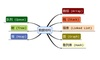数据结构 订阅